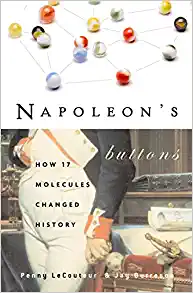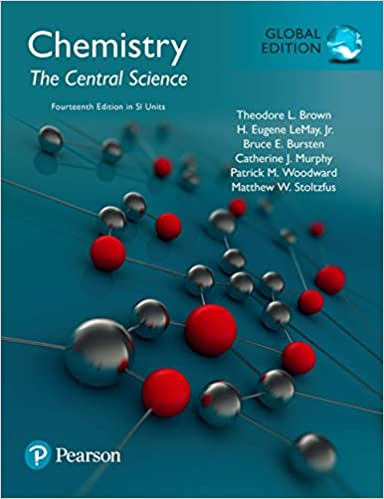# Ideal Gas Law

The Ideal Gas Law is PV = nRT, where P is pressure, V is volume, n is number of moles, T is temperature, and R is the Ideal Gas Constant. The Ideal Gas Law works in most situations to predict the behavior of gases - as long as the temperature isn’t too low, or the pressure too high, you can use the ideal gas law. It does involve some assumptions to make the math work - including assuming that collisions are perfectly elastic, and that the particles of gas are not attracted to each other. In this video, we talk about the circumstances where you can use the Ideal Gas Law, how it’s related to other gas laws (Boyle’s Law, Charles’s Law, and Avogadro’s Law) and then we work through 5 example problems in full.

For a gas, pressure and volume are indirectly proportional. When one goes up, the other goes down. Meanwhile, pressure and temperature are directly proportional—if you increase one, the other also increases. Volume and temperature are also directly proportional. And the number of moles are also directly proportional to the volume the gas occupies, as well as how much pressure it exerts. You can combine all of this information together in the Ideal Gas Law, which can be written mathematically as

PV = nRT

P = pressure

V= Volume

n = number of moles of gas

R = the Ideal Gas Constant, and

T = Temperature in Kelvin.

In this video, we talk about the circumstances where you can use the Ideal Gas Law, how it’s related to other gas laws (Boyle’s Law, Charles’s Law, and Avogadro’s Law) and then we work through 5 example problems in full. If you need more practice for a test, we have a Gas Laws Practice Test available for purchase.

Video: Ideal Gas Law

Handout: Video Notes Worksheet